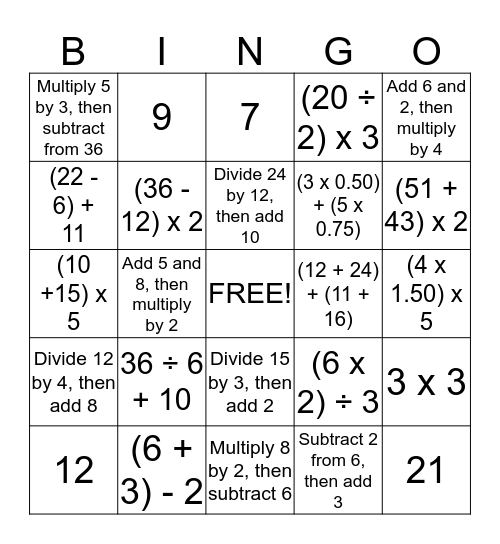# Math BingoThis bingo card has a free space and 24 words: 36 ÷ 6 + 10, (6 + 3) - 2, Divide 24 by 12, then add 10, 12, Add 6 and 2, then multiply by 4, Multiply 5 by 3, then subtract from 36, (22 - 6) + 11, 9, (12 + 24) + (11 + 16), Divide 15 by 3, then add 2, (3 x 0.50) + (5 x 0.75), (10 +15) x 5, (6 x 2) ÷ 3, 3 x 3, (36 - 12) x 2, Subtract 2 from 6, then add 3, (4 x 1.50) x 5, 7, (51 + 43) x 2, Add 5 and 8, then multiply by 2, 21, Divide 12 by 4, then add 8, (20 ÷ 2) x 3 and Multiply 8 by 2, then subtract 6.

## Play Online